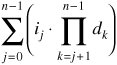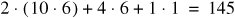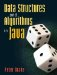# Contiguous Representation of Multidimensional Arrays

Recall from Figure 2-7 that a multidimensional array in Java is normally represented as an array of arrays. It is also possible to represent such an array as a single one-dimensional array (Figure 12-16). The rows are placed one right after another in memory, so this is called a contiguous representation.

Figure 12-16. A multidimensional array can be represented as an array of arrays (left) or as a single one-dimensional array (right).The only challenge here is determining which elements correspond in the two arrays. This is resolved with a simple formula. The element at position <r, c> in the array of arrays is at position r·w + c in the contiguous array, where w is the width of the array (number of columns). The term r·w gets us to the beginning of the right row. Adding c then gets us to the right position within that row.

This formula can be generalized to higher dimensions. Suppose we have an n-dimensional array with dimensions d0, d1, ..., dn1. The element at position <i0, i1, ..., in1> is found at index:The second large symbol above is an upper-case Greek letter pi. It indicates the product of many factors in the same way that an upper-case sigma indicates the sum of many terms. Pi stands for "product," sigma for "sum."

For example, in a 8 x 10 x 6 array, the element at position <2, 4, 1> is at index:This conversion seems like a lot of work. Is it worth the effort? Yes and no.

There are some advantages to this representation. It saves some space and time by eliminating references. By ensuring that all of the data are in one contiguous block of memory, it also ensures good cache performance. Finally, traversing every element of the array becomes slightly simplerit's a single for loop, rather than one nested loop for each dimension.

On the other hand, none of these advantages is huge. The number of references followed to reach a particular element in an array of arrays representation is the same as the number of dimensions. High-dimensional arrays are rare. If we allocate an array of arrays all at once (as we usually do), it is likely to all be placed in the same area of memory, so cache performance is not an issue. Finally, a few nested for loops may be less complicated than the conversion between coordinate systems.

It is usually just as well to use the array-of-arrays representation and let the compiler handle the coordinates. Still, the idea of contiguous representation can be useful, as we'll see in the next example and again in Section 14.1.

Example: Tic Tac Toe Revisited

Recall the game of Tic Tac Toe from Figure 10-25. Our implementation in Section 10.3 used an array of arrays of characters to represent the board. We will now write a different version, using the ideas of bit vectors and contiguous representation from this chapter.

We interpret the board as a set of nine squares, numbered 0 through 8. It can therefore be represented contiguously by an array of length 9. We could use a contiguous array of chars, but we go one step farther: we use bit vectors of length 9.

There is one bit vector for the squares occupied by X and another for the squares occupied by O. If we want to know which squares are occupied by either player (to determine whether a move is legal), we take the union (bitwise or) of these two bit vectors.

The code for the new program is given in Figure 12-17

Figure 12-17. The Tic Tac Toe program using bit vectors.

 ``` 1 import java.util.Scanner; 2 3 /** The game of Tic Tac Toe. */ 4 public class TicTacToe { 5 6 /** For reading from the console. */ 7 public static final Scanner INPUT = new Scanner(System.in); 8 9 /** Bit vector of squares occupied by X. */ 10 private int xSquares; 11 12 /** Bit vector of squares occupied by O. */ 13 private int oSquares; 14 15 /** Bit vector of all nine squares. */ 16 private int allSquares; 17 18 /** Bit vectors of winning triples of squares. */ 19 private int[] winningLines; 20 21 /** The board is initially empty. */ 22 public TicTacToe() { 23 xSquares = 0; 24 oSquares = 0; 25 allSquares = (1 << 9) - 1; 26 winningLines = new int; ``` 27 winningLines = 1 | (1 << 1) | (1 << 2); // Top row 28 winningLines = winningLines << 3; // Middle row 29 winningLines = winningLines << 3; // Bottom row 30 winningLines = 1 | (1 << 3) | (1 << 6); // Left column 31 winningLines = winningLines << 1; // Middle column 32 winningLines = winningLines << 1; // Right column 33 winningLines = 1 | (1 << 4) | (1 << 8); // Diagonal 34 winningLines = (1 << 2) | (1 << 4) | (1 << 6); // Diagonal 35 } 36 37 /** Return true if the game is over. */ 38 public boolean gameOver() { 39 if (score() != 0) { 40 return true; 41 } 42 return (xSquares | oSquares) == allSquares; 43 } 44 45 /** Return the value of the current position if it is O's turn. */ 46 protected int minimaxForO() { 47 int score = score(); 48 if (gameOver()) { 49 return score; 50 } 51 int bestScore = 2; 52 int occupied = xSquares | oSquares; 53 for (int move = 1; move < allSquares; move <<= 1) { 54 if ((occupied & move) == 0) { 55 oSquares |= move; // Play the move 56 score = minimaxForX(); 57 if (score < bestScore) { 58 bestScore = score; 59 } 60 oSquares ^= move; // Unplay the move 61 } 62 } 63 return bestScore; 64 } 65 66 /** Return the value of the current position if it is X's turn. */ 67 protected int minimaxForX() { 68 int score = score(); 69 if (gameOver()) { 70 return score; 71 } 72 int bestScore = -2; 73 int occupied = xSquares | oSquares; 74 for (int move = 1; move < allSquares; move <<= 1) { 75 if ((occupied & move) == 0) { 76 xSquares |= move; // Play the move 77 score = minimaxForO(); 78 if (score > bestScore) { 79 bestScore = score; 80 } 81 xSquares ^= move; // Unplay the move 82 } 83 } 84 return bestScore; 85 } 86 87 /** Play one game. */ 88 public void play() { 89 char player = 'X'; 90 for (int move = 0; move < 9; move++) { 91 if (gameOver()) { 92 return; 93 } 94 if (player == 'X') { 95 playBestMove(); 96 player = 'O'; 97 } else { 98 System.out.println(this); 99 System.out.print("Enter move (0-8): "); 100 int index = INPUT.nextInt(); 101 oSquares |= 1 << index; 102 player = 'X'; 103 } 104 } 105 } 106 107 /** Find the best move for X and play it on the board. */ 108 protected void playBestMove() { 109 int score; 110 int bestScore = -2; 111 int bestMove = -1; 112 int occupied = xSquares | oSquares; 113 for (int move = 1; move < allSquares; move <<= 1) { 114 if ((occupied & move) == 0) { 115 xSquares |= move; // Play the move 116 score = minimaxForO(); 117 if (score > bestScore) { 118 bestScore = score; 119 bestMove = move; 120 } 121 xSquares ^= move; // Unplay the move 122 } 123 } 124 xSquares |= bestMove; // Play the move 125 } 126 127 /** Return 1 if X has won, -1 if O has won, and 0 otherwise. */ 128 public int score() { 129 for (int line : winningLines) { 130 if ((xSquares & line) == line) { 131 return 1; 132 } 133 if ((oSquares & line) == line) { 134 return -1; 135 } 136 } 137 return 0; 138 } 139 140 public String toString() { 141 String result = ""; 142 int column = 0; 143 for (int square = 1; square < allSquares; square <<= 1) { 144 if ((xSquares & square) != 0) { 145 result += 'X'; 146 } else if ((oSquares & square) != 0) { 147 result += 'O'; 148 } else { 149 result += '.'; 150 } 151 column++; 152 if (column % 3 == 0) { result += " "; } 153 } 154 return result; 155 } 156 157 // See Figure 10-27 for the main() method 158 }

When we need to iterate through the squares on the board, we use a for loop of the form:

```for (int move = 1; move < allSquares; move <<= 1) { ... }
```

Within the body of such a loop, move is the bit vector ending in 000000001 on the first pass, 000000010 on the second pass, and so on.

Exercises

 12.15 A contiguous array of length r·c is used to represent a two-dimensional array with r rows and c columns. Give formulae for finding the row and column of element i in the contiguous array. 12.16 In a triangular array of width w, the first row has w columns, the second row w 1 columns, and so on down to the last row, which has one column. This can be represented as one contiguous array. Devise a formula for finding the index of the element in row r, column c. 12.17 Would a contiguous representation work well in general for ragged arrays? Explain. 12.18 Explain lines 25 and 120 of the Tic Tac Toe program in Figure 12-17.Data Structures and Algorithms in Java
ISBN: 0131469142
EAN: 2147483647
Year: 2004
Pages: 216
Authors: Peter Drake